# 主成分分析PCA原理以及代码实现

### 主成分分析

PCA（Principal Component Analysis）是一种常用的数据分析方法。PCA通过线性变换将原始数据变换为一组各维度线性无关的表示，可用于提取数据的主要特征分量，常用于高维数据的降维。

## 数据降维问题

PCA是一种具有严格数学基础并且已被广泛采用的降维方法。

## 基变换

1.向量A和B(模为1)的内积表示的是向量A在B上的投影长度。那么将一个向量与新的基做内积，结果则表示该向量在新的基下的坐标。
2.将新选定的基表示成矩阵形式，与原向量相乘，就得到了原向量在新选定的基所表示的空间（或坐标系）中的坐标表示了。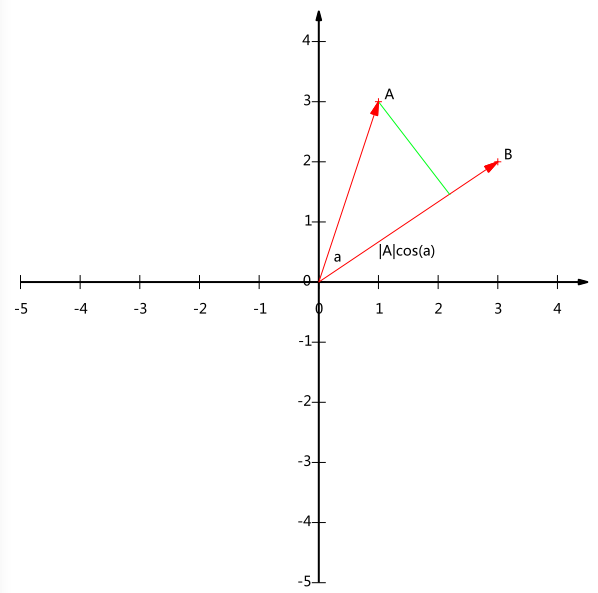### 基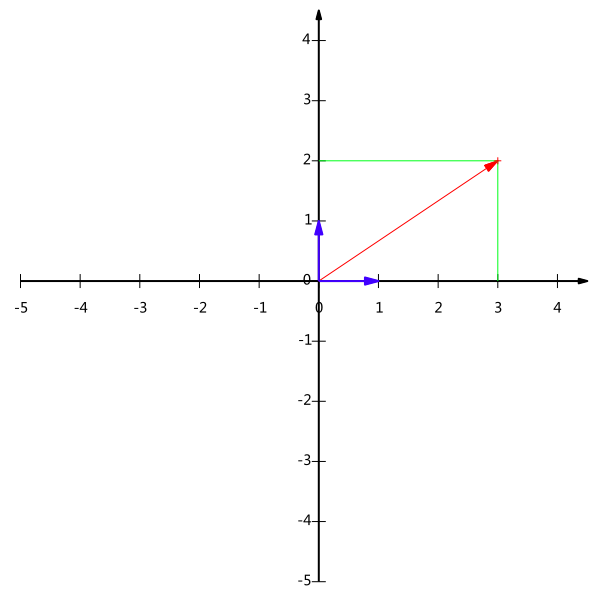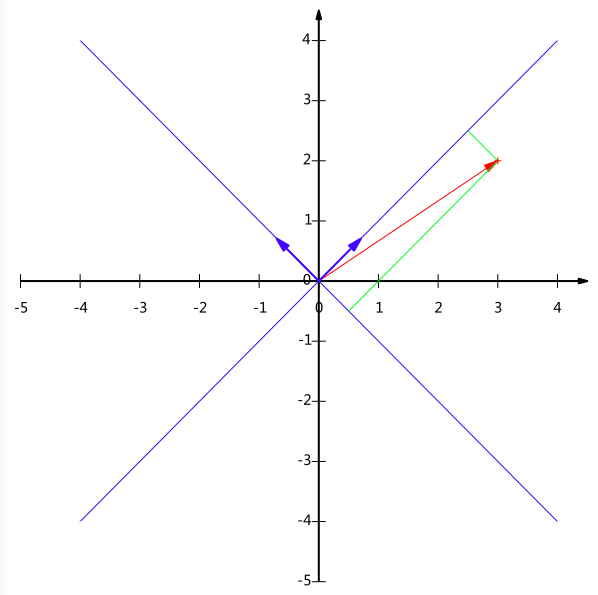### 基变换矩阵表示## 协方差矩阵及优化目标

1.怎样选定这组基用于数据降维？（目标）
（1）首先将数据变换到选定基上后，数据的方差要大，尽量分散。
（2）各个基要正交（表示的信息要不相关，若单纯按方差选定基，则选定的基存在很大的相关性）
2.怎么做?怎么找这组正交基？ （做法）
（1）协方差矩阵可以很好地表示各个特征的方差和不同特征之间的协方差
（2）按目标要求需要将协方差矩阵除去对角线元素化为0，对角线元素按从大到小排列。协方差矩阵对角化。
（3）对角化后按特征值大小排列，选定前k个最大的特征值对应的特征向量作为降维后的新基。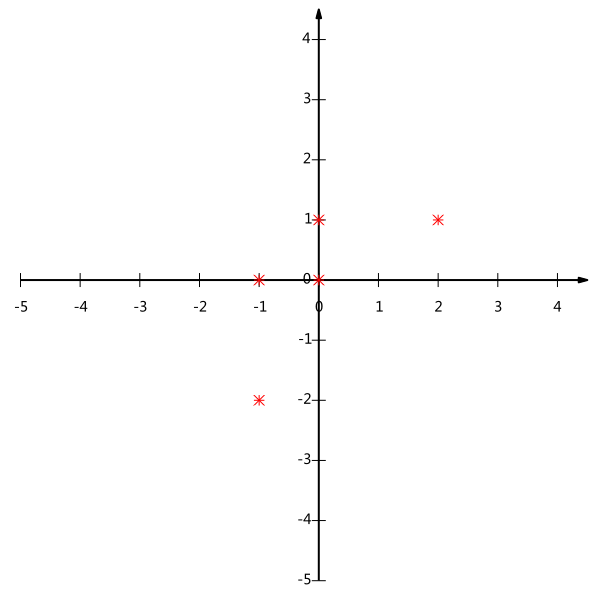### 方差### 协方差### 协方差矩阵### 协方差矩阵对角化1）实对称矩阵不同特征值对应的特征向量必然正交。
2）设特征向量λ重数为r，则必然存在r个线性无关的特征向量对应于λ，因此可以将这r个特征向量单位正交化。P是协方差矩阵的特征向量单位化后按行排列出的矩阵，其中每一行都是C的一个特征向量。如果设P按照Λ中特征值的从大到小，将特征向量从上到下排列，则用P的前K行组成的矩阵乘以原始数据矩阵X，就得到了我们需要的降维后的数据矩阵Y

## PCA算法

1）将原始数据按列组成n行m列矩阵X
2）将X的每一行（代表一个属性字段）进行零均值化，即减去这一行的均值
3）求出协方差矩阵4）求出协方差矩阵的特征值及对应的特征向量
5）将特征向量按对应特征值大小从上到下按行排列成矩阵，取前k行组成矩阵P
6）Y=PX即为降维到k维后的数据

## 实例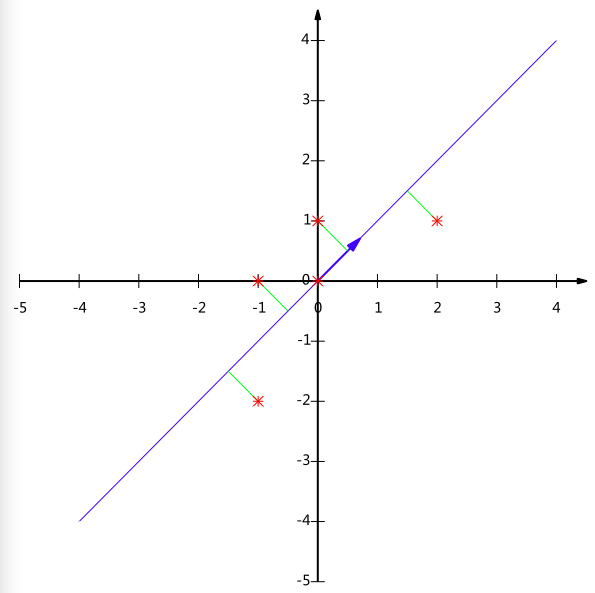## 代码实现

import numpy as np
import matplotlib.pyplot as plt
from sklearn.decomposition import PCA
import pandas as pd
%matplotlib inline


data=pd.read_csv('F:\jupyter notebook workspace\data\\iris.csv')
x=data.iloc[:,0:4]
y=data.iloc[:,4]


x.columns=['sepal_length','sepal_width','petal_length','petal_width']
labels=['iris_setosa','iris_versicolor','iris_virginica']


#根据值获取索引
y_0_index=y[y.values==0].index
y_1_index=y[y.values==1].index
y_2_index=y[y.values==2].index

x_0=[];x_1=[];x_2=[]
for i,j,k in zip(y_0_index,y_1_index,y_2_index):
x_0.append(x.values[i])
x_1.append(x.values[j])
x_2.append(x.values[k])


x_0=np.array(x_0);x_1=np.array(x_1);x_2=np.array(x_2)
iris_setosa=plt.scatter(x_0[:,0],x_0[:,1],color='blue')
iris_versicolor=plt.scatter(x_1[:,0],x_1[:,1],color='red')
iris_virginica=plt.scatter(x_2[:,0],x_2[:,1],color='green')
plt.xlabel('sepal_length')
plt.ylabel('sepal_width')
plt.legend((iris_setosa, iris_versicolor, iris_virginica), ('iris_setosa', 'iris_versicolor', 'iris_virginica'))
plt.savefig('F:\jupyter notebook workspace\photo\PCA\\origin.jpg')pca=PCA(n_components=2)     #加载PCA算法，设置降维后主成分数目为2
reduced_x=pca.fit_transform(x)#对样本进行降维


[[-2.68412563,  0.31939725],
[-2.71414169, -0.17700123],
[-2.88899057, -0.14494943],
[-2.74534286, -0.31829898],
[-2.72871654,  0.32675451],
[-2.28085963,  0.74133045],
[-2.82053775, -0.08946138],
.......]


reduced_x_0=[];reduced_x_1=[];reduced_x_2=[]
for i,j,k in zip(y_0_index,y_1_index,y_2_index):
reduced_x_0.append(reduced_x[i])
reduced_x_1.append(reduced_x[j])
reduced_x_2.append(reduced_x[k])


reduced_x_0=np.array(reduced_x_0);reduced_x_1=np.array(reduced_x_1);reduced_x_2=np.array(reduced_x_2)
iris_setosa=plt.scatter(reduced_x_0[:,0],reduced_x_0[:,1],color='blue')
iris_versicolor=plt.scatter(reduced_x_1[:,0],reduced_x_1[:,1],color='red')
iris_virginica=plt.scatter(reduced_x_2[:,0],reduced_x_2[:,1],color='green')
plt.legend((iris_setosa, iris_versicolor, iris_virginica), ('iris_setosa', 'iris_versicolor', 'iris_virginica'))
plt.xlabel('Principal Component1')
plt.ylabel('Principal Component2')
plt.savefig('F:\jupyter notebook workspace\photo\PCA\pca.jpg')## 总结

PCA本质上是将方差最大的方向作为主要特征，并且在各个正交方向上将数据“离相关”，也就是让它们在不同正交方向上没有相关性。

04-23
03-261万+07-13657
04-095万+
09-14278
05-084785
02-19
01-274828
03-232万+
09-07
04-20133
06-24204
08-01
08-19890
08-139万+
09-23548
03-13491
12-07
02-221065点击重新获取扫码支付余额充值